Courses

# Theory & Procedure, Figure of Merit of a Galvanometer Class 12 Notes | EduRev

## JEE : Theory & Procedure, Figure of Merit of a Galvanometer Class 12 Notes | EduRev

The document Theory & Procedure, Figure of Merit of a Galvanometer Class 12 Notes | EduRev is a part of the JEE Course Physics Class 12.
All you need of JEE at this link: JEE

Objective

To determine the resistance of a galvanometer by half deflection method and to find its figure of merit.

Theory

Galvanometer

A galvanometer is a device used to detect feeble electric currents in a circuit. It consists of a coil suspended between the poles of a powerful magnet. As current passes through the coil, it deflects. It can be detected from the deflection on galvanometer needle. The deflection is proportional to the current passed through it.

Resistance of galvanometer by half deflection method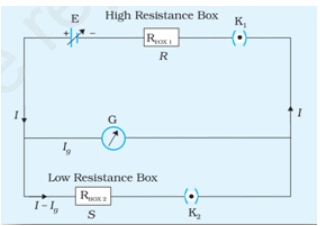Here, current will flows through th circuit when key k1 is closed and k2 is open. The current flowing through the galvanometer is proportional to the deflection in it.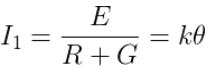Where, E - emf f the cell

R - resistance from the resistance box
G - galvanometer resistance for current I
θ - galvanometer deflection for current I
k – proportionality constant.

When k2 is closed and by adjusting the shunt resistance S, we can make galvanometer deflection as θ/2.

Then the current in the circuit is ;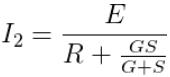Now, a fraction, S/ (G+S) of the current in the circuit is flows through the galvanometer, which is given by,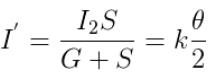Now, from the above relations, we can get the resistance of the given galvanometer as,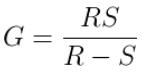Figure of merit of a galvanometer

Figure of merit is in general is the numerical value representing the degree of effectiveness or efficiency of an instrument approximated by different estimation techniques.

The figure of merit of a galvanometer is the current required to produce a deflection of one division in the galvanometer scale. It is represented by the letter k, and is given as,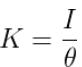or  ,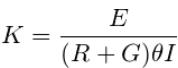Learning Outcomes

• Students understand the various components used in the experiment.
• Students learn the concept, ‘figure of merit’.
• Students are able to construct circuits based on circuit diagrams.

Materials Required

• A weston type galvanometer
• A battery or battery eliminator
• Two resistance boxes
• Two one-way keys
• Connecting wires

Real Lab Procedure

Resistance of galvanometer by half deflection method :

• Arrange the components on a table and connect them as per the circuit diagram.
• Make sure that plugs of the resistance boxes are tight.
• Take out a high resistance from the resistance box 1 and insert the key k1.
• Adjust the resistance from this resistance box to get maximum galvanometer deflection.
• Note the deflection and record it as θ in the tubular column.
• Insert the key k2 also, without changing the value on the resistance box.
• Now, adjust the resistance from the low resistance box such that galvanometer shows deflection which is exactly half of the previous reading.
• Record the value of low resistance box.
• We can repeat the experiment by changing the value of high resistance R and adjusting low resistance S.
• The resistance of the given galvanometer can be calculated each time by using the relation G= RS/(R-S).

Figure of merit of the galvanometer :

• Release key k2 from the connection. Insert key k1.
• Adjust the value of R such that the galvanometer shows a certain deflection.
• Record the observations in a tabular column.
• Repeat the experiment by changing the value of R and note the galvanometer deflection each time.
• We can find the figure of merit of the galvanometer by using the equation, k= E/(R+G)θ.

Simulator Procedure (as performed through the Online Labs)

• Select the ‘Galvanometer’ form the drop down list.
• To see the circuit diagram, click on the ‘Show circuit diagram’ check box seen inside the simulator window.
• Connections can be made as seen in the circuit diagram by clicking and dragging the mouse from one connecting terminal to the other connecting terminal of the devices to be connected.
• Once all connections are made, click and drag key 1 to insert it into the switch.
• You can select the desired large resistance from the resistance box.
• The galvanometer shows a deflection.
• Now click and drag key 2 to insert it into the switch without changing the value of the resistance.
• Select the resistance from the small resistance box such that the galvanometer shows deflection which is exactly half of the previous reading.
• To see the result, click on the ‘Show result’ check box.
• To redo the experiment, click on the ‘Reset’ button.

Calculations

Calculate the value of G in each case and record it in the tabular column. The mean of these calculated values will give the resistance of the given galvanometer.

Calculate the value of k in each case and record it in the tabular column. The mean of these calculated values will give the figure of merit of the given galvanometer.

Results

The resistance of the given galvanometer, G     =--------- Ω
The figure of merit of the given galvanometer, k =---------- Amp / div.

Offer running on EduRev: Apply code STAYHOME200 to get INR 200 off on our premium plan EduRev Infinity!

## Physics Class 12

204 videos|288 docs|125 tests

,

,

,

,

,

,

,

,

,

,

,

,

,

,

,

,

,

,

,

,

,

,

,

,

;